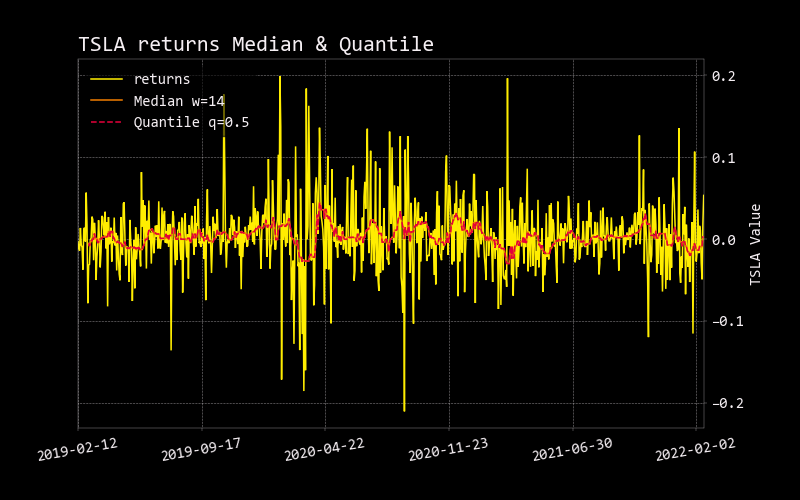# quantile

The quantiles are values which divide the distribution such that there is a given proportion of observations below the quantile. For example, the median is a quantile. The median is the central value of the distribution, such that half the points are less than or equal to it and half are greater than or equal to it. By default, q is set at 0.5, which effectively is median. Change q to get the desired quantile (0q1).

### Usage​

quantile [-w N_WINDOW] [-q F_QUANTILE]

## Parameters​

NameParameterDescriptionDefaultOptionalChoices
n_window-w --windowwindow length14TrueNone
f_quantile-q --quantilequantile0.5TrueNone